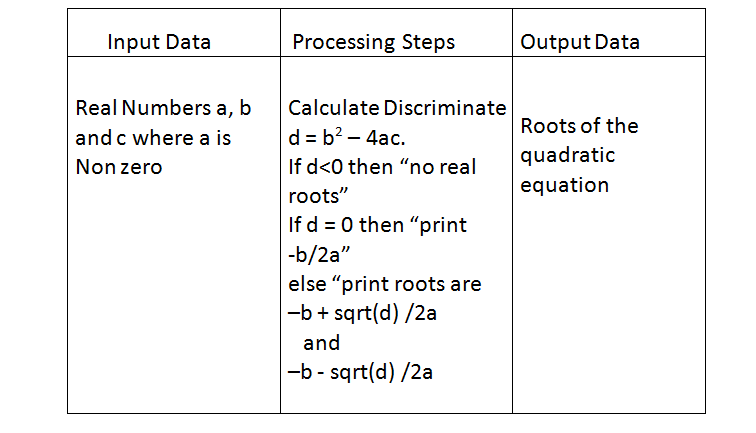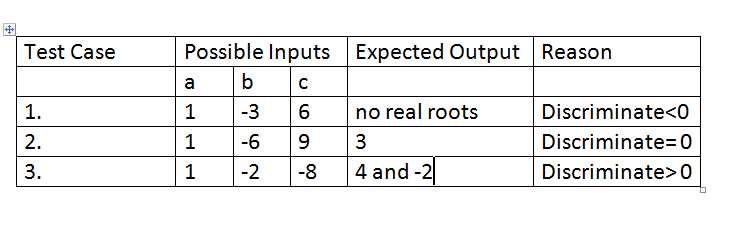# Question & Answer: Design an algorithm to find the real roots of a quadratic equation of the form ax2 + bx + c = 0, where a, b, and c are…..

Design an algorithm to find the real roots of a quadratic equation of the form ax2 + bx + c = 0, where ab, and c are real numbers, and a is nonzero.

Include:

Don't use plagiarized sources. Get Your Custom Essay on
Question & Answer: Design an algorithm to find the real roots of a quadratic equation of the form ax2 + bx + c = 0, where a, b, and c are…..
GET AN ESSAY WRITTEN FOR YOU FROM AS LOW AS \$13/PAGE

IPO Chart

Test Plan

Pseudocode Algorithm

IPO ChartTest PlanPseudocode Algorithm

1. let d = b * b – 4 * a * c
2. if d < 0 then
3. print “No real roots.”
4. else if d = 0 then
5. print “Root is: “; -b / (2 * a); “.”
6. else
7. print “Roots are: “; (-b + sqrt ( d )) / (2 * a); ” and “; (-b – sqrt ( d )) / (2 * a); “.”
8. end if